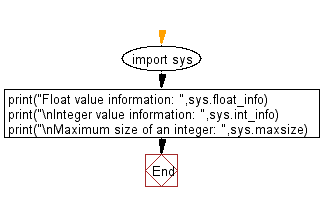﻿ Python: Determine the largest and smallest integers, longs, floats - w3resource# Python: Determine the largest and smallest integers, longs, floats

## Python Basic: Exercise-123 with Solution

Write a Python program to determine the largest and smallest integers, longs, floats.

Sample Solution:-

Python Code:

``````import sys
print("Float value information: ",sys.float_info)
print("\nInteger value information: ",sys.int_info)
print("\nMaximum size of an integer: ",sys.maxsize)
```
```

Sample Output:

```Float value information: sys.float_info(max=1.7976931348623157e+308, max_exp=1024, max_10_exp=308, min=2.2250
738585072014e-308, min_exp=-1021, min_10_exp=-307, dig=15, mant_dig=53, epsilon=2.220446049250313e-16, radix=2
, rounds=1)

Integer value information: sys.int_info(bits_per_digit=30, sizeof_digit=4)

Maximum size of an integer: 9223372036854775807
```

Flowchart:Python Code Editor:

Have another way to solve this solution? Contribute your code (and comments) through Disqus.

What is the difficulty level of this exercise?

Test your Python skills with w3resource's quiz

﻿

## Python: Tips of the Day

Unpack variables from iterable:

```# One can unpack all iterables (tuples, list etc)
>>> a, b, c = 1, 2, 3
>>> a, b, c
(1, 2, 3)

>>> a, b, c = [1, 2, 3]
>>> a, b, c
(1, 2, 3)
```